# Resources to Learn Machine Learning

## Mathematical Prerequisites

Machine learning theory makes extensive use of mathematics. This might put you off if you are not familiar with the requisite mathematical topics and notation.

However, keep in mind, that it is possible to learn and apply machine learning algorithms without understanding all the mathematical details. Modern libraries such as scikit-learn make it very easy to fit models to data, such as linear regression, to make some predictions. For more advanced models, for example, to use neural networks, you can use something like Keras.

My advice is to start playing around with models using some library and then fill in any mathematical knowledge gaps as required. The mathematical knowledge will help you to better understand how each machine learning algorithm works. You will thus be able to make an informed decision when choosing between different methods. It will also come in handy while debugging or trying to improve performance.

If, on the other hand, you want to have in-depth knowledge of machine learning and want to understand research papers in the field, then the mathematical knowledge is a requirement.

The mathematical topics you need to look into to get started with machine learning are probability and statistics, linear algebra and calculus. Information theory is another very important topic if you really want to go deep into machine learning theory.

### Mathematics Course

#### By Salman Khan - Khan AcademyIf your mathematical knowledge is a little bit rusty or need a gentle introduction to the basics of probability, statistics, linear algebra and calculus, the brief one-to-one lectures and interactive exercises at Khan Academy - Math will help you get up to speed real quick.

### Statistics 110: Probability

#### By Joe Blitzstein - Harvard University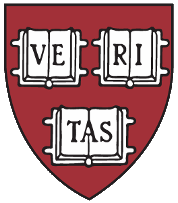In these 34 video lectures you will get an introduction to probability including topics such as sample spaces and events, conditioning, Bayes' Theorem, distributions, both univariate and multivariate, markov chains, and limit theorems, i.e. the law of large numbers and the central limit theorem. The handouts and practice problems with solutions are also available.

### 18-06sc Linear Algebra

#### By Gilbert Strang - MITAs part of the MIT OpenCourseWare, this Linear Algebra course is delivered by eminent professor Gilbert Strang, an excellent lecturer.

### 18-01sc Single Variable Calculus

#### By David Jerison - MITAs part of the MIT OpenCourseWare, this single variable calculus course covers differentiation and integration of functions of one variable.

### 18-02sc Multivariable Calculus

#### By Denis Auroux - MITAs part of the MIT OpenCourseWare, this multivariable calculus course follows on from where 18-01sc Single Variable Calculus left, to cover differentiation and integration of functions of more than one variable.The video lectures by mathematicalmonk assume a certain level of mathematical knowledge since they are intended for senior undergraduates or graduate students. Having said that, the topics are introduced at a gentle pace and are very well explained, so do give them a try. I would suggest starting off with the probability primer series and if you really want to study machine learning in detail you should also view the information theory series of lectures.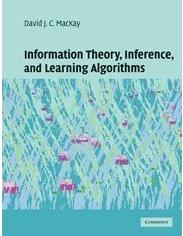Information theory, founded by Claude Shannon, is a fundamental theory underpinning many modern technologies amongst which data compression and machine learning. I think the best way to learn information theory is through this book by the late David MacKay. If you want to watch lectures go to the Information Theory and Pattern Recognition course page where a series of 16 lectures is available.

## Machine Learning Theory

### An Introduction to Statistical Learning with Applications in R

#### By Gareth James, Daniela Witten, Trevor Hastie and Robert Tibshirani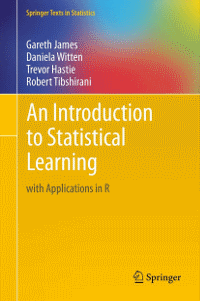This book is really good if you are getting started, and is available online for free, get it here. It uses the R statistical language, however, if you are more into Python, Jordi Warmenhoven was kind enough to provide a GitHub repo implementing some of the book code in Python.

### The Elements of Statistical Learning: Data Mining, Inference, and Prediction

#### By Trevor Hastie, Robert Tibshirani and Jerome Friedman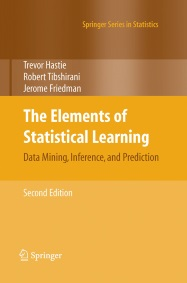This book is also by Hastie and Tibshirani, amongst others, but is much more mathematical than An Introduction to Statistical Learning with Applications in R. In fact, this was the original book on the subject by the authors and the introduction book was released later to cater for a less mathematically knowledgeable audience that prefers a more hands on approach. This book is also available for free, get it here.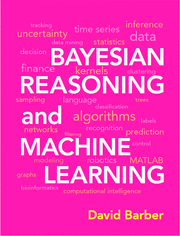This book covers a lot of material, from probabilistic graphical models, Bayesian methods, supervised and unsupervised methods, Gaussian processes and mixture models, all the way to dynamical systems for timeseries analysis and predictions. Although the mathematical content in this book is introduced gradually and kept to the minimum possible, an undergraduate level of knowledge in statistics, linear algebra and calculus are assumed. I would say it sits somewhere in between the An Introduction to Statistical Learning with Applications in R book and the more maths heavy books such as Kevin Murphy's Machine Learning: A Probabilistic Approach and Christopher M. Bishop's book Pattern Recognition and Machine Learning, which is also available for free.

### Machine Learning Lecture Series

#### By mathematicalmonkJust like the probability primer and information theory video lectures by mathematicalmonk, the machine learning lecture series are a gem. They start off explaining the difference between supervised and unsupervised machine learning, moving on to k-nearest neighbour classification, trees, bootstrapping, random forests, maximum likelihood estimation (MLE) and maximum a posteriori (MAP) estimation, all the way to bayesian methods, graphical models, and hidden markov models. There are over 100 lectures of not more than 15 minutes each.

### Machine Learning Course

#### By Andrew Ng - Coursera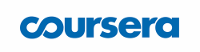If I am not mistaken this is the first massive open online course (MOOC) on machine learning delivered by one of the finest minds in the field, Andrew Ng, professor at Stanford University. Sign up for free on Coursera to enroll on the machine learning course. The material is explained very well and although there is some mathematical notation involved every now and then, it is kept to a minimum, with the emphasis being more on getting an intuition for how the algorithms presented work.

### CS229 - Machine Learning

#### By Andrew Ng - Stanford UniversityIf the machine learning course delivered by Andrew Ng on Coursera left you wanting to learn more about the mathematical underpinnings, head to the machine learning lecture collection delivered by Andrew Ng at Stanford University.

## Neural Networks - Deep Learning

### Neural Network Class

#### By Hugo Larochelle - Université de SherbrookeHugo Larochelle's neural network class covers a lot of material starting from simple feedforward neural networks and how to train them, then moving on to conditional random fields and restricted Boltzmann machines, and finishing off with deep neural networks with applications in computer vision and natural language processing.

### CS231n - Convolutional Neural Networks for Visual Recognition

#### By Fei-Fei Li, Andrej Karpathy and Justin Johnson - Stanford UniversityCS231n focuses on deep learning for computer vision applications, specifically convolutional neural networks. If you want to supplement the lectures with notes go to the CS231n notes page.CS224n is a merger of Stanford's previous CS224n Natural Language Processing course and CS224d Deep Learning for Natural Language Processing, which I still list below. This series of lectures was delivered during winter 2021, and through it you will learn to implement, train, visualize and invent your own neural network models. The course introduces cutting-edge research in deep learning applied to NLP, including all models previously covered in CS224d, along with recent models using a memory component. If you prefer to read notes and other suggested readings go to the CS224n syllabus.CS224d focuses on deep neural network techniques applied to natural language processing, covering topics such as recurrent neural networks, long short-term memory (LSTM) architecture, recursive neural networks, convolutional neural networks for sentence classification and also dynamic memory networks. If you prefer to read notes and other suggested readings go to the CS224d syllabus page.

Tags:

Categories:

Updated: